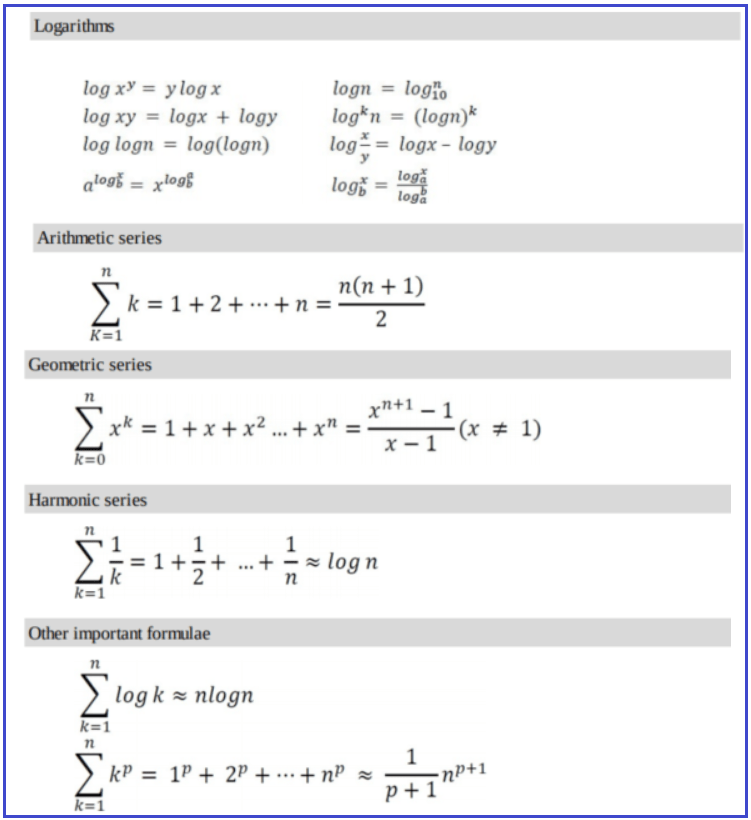# Properties of Asymptotic Notations

## Properties of Asymptotic Notations in Data Structure and Algorithms

In this article, I am going to discuss the Properties of Asymptotic Notations in Data Structure and Algorithms. Please read our previous article where we discussed Asymptotic Notations. As part of this article, we are going to discuss the following Asymptotic Notations Properties.

1. General Properties
2. Reflexive Properties
3. Transitive Properties
4. Symmetric Properties
5. Transpose Symmetric Properties
##### Properties of Asymptotic Notations:

As we have gone through the definition of these three notations (Big-O, Omega-Q, Theta-Θ) in our previous article. Now let’s discuss some important properties of those notations.

##### General Properties:

If f(n) is O(g(n)) then a*f(n) is also O(g(n)) ; where a is a constant.

Example:
f(n) = 2n²+5 is O(n²)
then 7*f(n) = 7(2n²+5)
= 14n²+35 is also O(n²)

Similarly, this property satisfies both Θ and Ω notation. We can say
If f(n) is Θ(g(n)) then a*f(n) is also Θ(g(n)); where a is a constant.
If f(n) is Ω (g(n)) then a*f(n) is also Ω (g(n)); where a is a constant.

##### Reflexive Properties:

If f(n) is given then f(n) is O(f(n)).
Example: f(n) = n² ; O(n²) i.e O(f(n))

Similarly, this property satisfies both Θ and Ω notation. We can say
If f(n) is given then f(n) is Θ(f(n)).
If f(n) is given then f(n) is Ω (f(n)).

##### Transitive Properties :

If f(n) is O(g(n)) and g(n) is O(h(n)) then f(n) = O(h(n)) .
Example: if f(n) = n , g(n) = n² and h(n)=n³
n is O(n²) and n² is O(n³) then n is O(n³)

Similarly this property satisfies for both Θ and Ω notation. We can say
If f(n) is Θ(g(n)) and g(n) is Θ(h(n)) then f(n) = Θ(h(n)) .
If f(n) is Ω (g(n)) and g(n) is Ω (h(n)) then f(n) = Ω (h(n))

##### Symmetric Properties :

If f(n) is Θ(g(n)) then g(n) is Θ(f(n)) .
Example: f(n) = n² and g(n) = n² then f(n) = Θ(n²) and g(n) = Θ(n²)
This property only satisfies for Θ notation.

##### Transpose Symmetric Properties :

If f(n) is O(g(n)) then g(n) is Ω (f(n)).
Example: f(n) = n , g(n) = n² then n is O(n²) and n² is Ω (n)
This property only satisfies for O and Ω notations.

##### Some More Properties :

1. If f(n) = O(g(n)) and f(n) = Ω(g(n)) then f(n) = Θ(g(n))
2. If f(n) = O(g(n)) and d(n)=O(e(n))
then f(n) + d(n) = O( max( g(n), e(n) ))

##### Example: f(n) = n i.e O(n)

d(n) = n² i.e O(n²)
then f(n) + d(n) = n + n² i.e O(n²)

3.If f(n)=O(g(n)) and d(n)=O(e(n))
then f(n) * d(n) = O( g(n) * e(n) )

##### Example: f(n) = n i.e O(n)

d(n) = n² i.e O(n²)
then f(n) * d(n) = n * n² = n³ i.e O(n³)

##### Commonly used Logarithms and Summations: